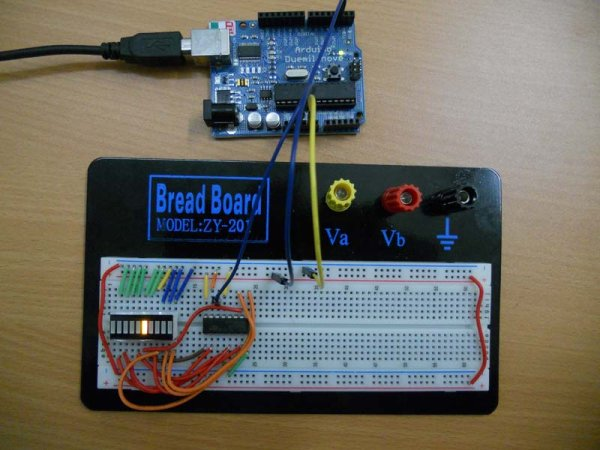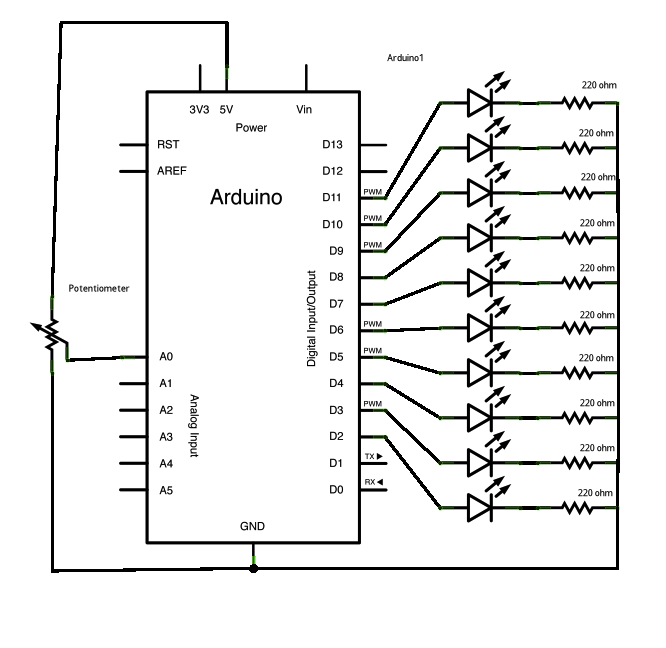# Arduino LED Bar Graph Code

The bar graph – a series of LEDs in a line, such as you see on an audio display – is a common hardware display for analog sensors.It’s made up of a series of LEDs in a row, an analog input like a potentiometer, and a little code in between. You can buy multi-LED bar graph displays fairly cheaply, like this one. This tutorial demonstrates how to control a series of LEDs in a row, but can be applied to any series of digital outputs.

This tutorial borrows from the For Loop and Arrays tutorial as well as the Analog Input tutorial.

The sketch works like this: first you read the input. You map the input value to the output range, in this case ten LEDs. Then you set up a for loop to iterate over the outputs. If the output’s number in the series is lower than the mapped input range, you turn it on. If not, you turn it off.

### Circuit

image developed using Fritzing. For more circuit examples, see the Fritzing project page

Schematic:### Code

```/*
LED bar graph

Turns on a series of LEDs based on the value of an analog sensor.
This is a simple way to make a bar graph display. Though this graph
uses 10 LEDs, you can use any number by changing the LED count
and the pins in the array.

This method can be used to control any series of digital outputs that

The circuit:
* LEDs from pins 2 through 11 to ground

created 4 Sep 2010
by Tom Igoe

This example code is in the public domain.

http://www.arduino.cc/en/Tutorial/BarGraph
*/```

### Hardware Required

• Arduino Board
• (1) LED bar graph display or 10 LEDs
• (10) 220 ohm resistors
• hook-up wire

For more detail: Arduino LED Bar Graph Code

#### This Post / Project can also be found using search terms:

• arduino bargraph code
• dc-10ewa arduino led bar
• arduino led bar
• bar graph code